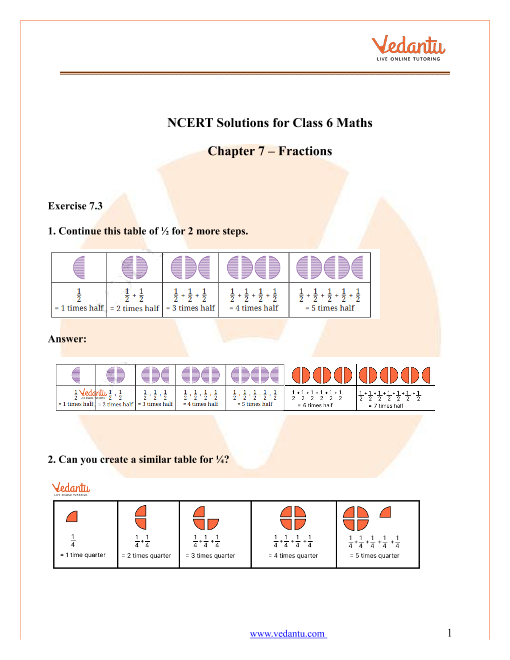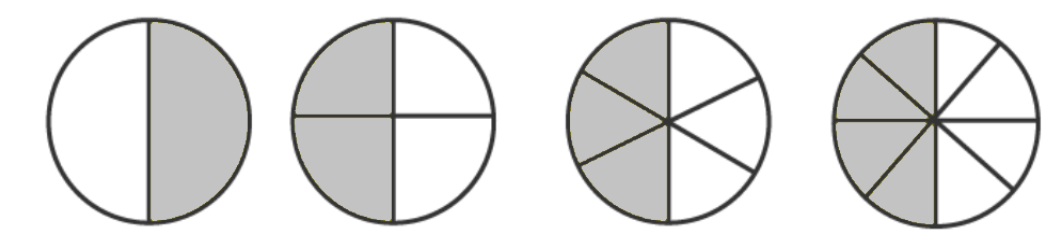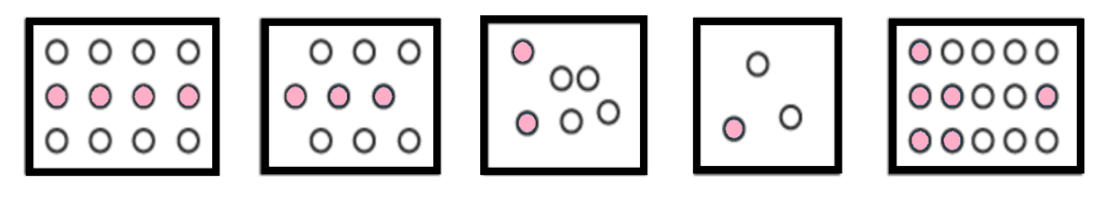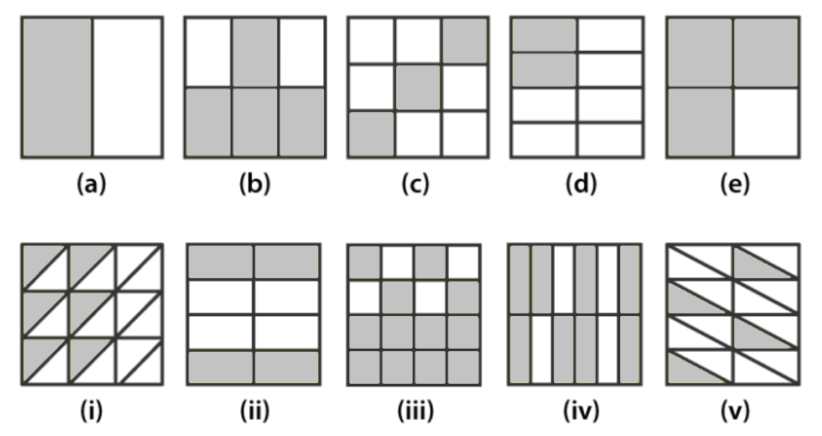# NCERT Solutions for Class 6 Maths Chapter 7 Fractions (Ex 7.3) Exercise 7.3

## NCERT Solutions for Class 6 Maths Chapter 7 Fractions (Ex 7.3) Exercise 7.3

Free PDF download of NCERT Solutions for Class 6 Maths Chapter 7 Exercise 7.3 (Ex 7.3) and all chapter exercises at one place prepared by an expert teacher as per NCERT (CBSE) books guidelines. Class 6 Maths Chapter 7 Fractions Exercise 7.3 Questions with Solutions to help you to revise complete Syllabus and Score More marks. Register and get all exercise solutions in your emails.

Every NCERT Solution is provided to make the study simple and interesting on Vedantu. Subjects like Science, Maths, English,Hindi will become easy to study if you have access to NCERT Solution for Class 6 Science , Maths solutions and solutions of other subjects.

Do you need help with your Homework? Are you preparing for Exams?
Study without Internet (Offline)Book your Free Demo session
Get a flavour of LIVE classes here at Vedantu## Access NCERT Solutions for Class 6 Mathematics Chapter 7- Fractions

Exercise 7.3

1. Write the fractions for the following. Are all these fractions equivalent?

a.Ans: Observe the first circle. It is divided into two parts out of which one part is shaded.

So, the fraction is $\dfrac{1}{2}$.

Now, Observe the second circle. It is divided into four parts out of which two parts are shaded. So, the fraction is  $\dfrac{2}{4}$. Thus, $\dfrac{{2}}{{4}}=\dfrac{1}{2}$.

Observe the third circle. It is divided into six parts out of which three parts are shaded. So, the fraction is  $\dfrac{3}{6}$. Thus, $\dfrac{{3}}{{6}}=\dfrac{1}{2}$.

Observe the fourth circle. It is divided into eight parts out of which four parts are shaded. So, the fraction is  $\dfrac{4}{8}$. Thus, $\dfrac{{4}}{{8}}=\dfrac{1}{2}$.

Hence, all the fractions are equivalent.

b.Ans: Observe the first figure. Four circles are shaded out of 12 circles. So, the fraction is $\dfrac{4}{12}$. Thus, $\dfrac{{4}}{{12}}=\dfrac{1}{3}$.

Observe the second figure. Three circles are shaded out of nine circles. So, the fraction is $\dfrac{3}{9}$. Thus, $\dfrac{{3}}{{9}}=\dfrac{1}{3}$

Observe the third figure. Two circles are shaded out of six circles. So, the fraction is $\dfrac{2}{6}$. Thus, $\dfrac{{2}}{{6}}=\dfrac{1}{3}$.

Observe the fourth figure. One circle is shaded out of three circles. So, the fraction is $\dfrac{1}{3}$.

Observe the fifth figure. Six circles are shaded out of fifteen circles. So, the fraction is $\dfrac{6}{15}$. Thus, $\dfrac{{6}}{{15}}=\dfrac{2}{5}$.

2. Write the fraction and pair up the equivalent fractions to each row.Ans:  Find the fractions of each part and then match the equivalent fractions.

In option (a), one part is shaded out of two parts. Thus, the fraction is $\dfrac{1}{2}$.

In option (b), four parts are shaded out of six parts. Thus, the fraction is $\dfrac{4}{6}$.

$\dfrac{{4}}{{6}}=\dfrac{2}{3}$

In option (c), three parts are shaded out of nine parts. Thus, the fraction is $\dfrac{3}{9}$.

$\dfrac{{3}}{{9}}=\dfrac{1}{3}$

In option (d), three parts are shaded out of four parts. Thus, the fraction is $\dfrac{2}{8}$.

$\dfrac{{2}}{{8}}=\dfrac{1}{4}$

In option (e), three parts are shaded out of four parts. Thus, the fraction is $\dfrac{3}{4}$.

In option (i), six parts are shaded out of eighteen parts. Thus, the fraction is $\dfrac{6}{18}$.

$\dfrac{{6}}{{18}}=\dfrac{1}{3}$

In option (ii), four parts are shaded out of eight parts. Thus, the fraction is $\dfrac{4}{8}$.

$\dfrac{{4}}{{8}}=\dfrac{1}{2}$

In option (iii), twelve parts are shaded out of sixteen parts. Thus, the fraction is$\dfrac{12}{16}$.

$\dfrac{{12}}{{16}}=\dfrac{3}{4}$

In option (iv), eight parts are shaded out of twelve parts. Thus, the fraction is $\dfrac{8}{12} =\dfrac{2}{3}$

In option (v), four parts are shaded out of sixteen parts. Thus, the fraction is $\dfrac{4}{16}$.

$\dfrac{{4}}{{16}}=\dfrac{1}{4}$

Thus, from the above observations the fractions can be matched as follows.

Option (a) matches with option (ii).

Option (b) matches with option (iv).

Option (c) matches with option (i).

Option (d) matches with option (v).

Option (e) matches with option (iii).

3. Replace $\boxed{}$ in each of the following by the correct number.

a. $\dfrac{2}{7} = \dfrac{8}{{\boxed{}}}$

Ans: Multiply the numerator and denominator by 4 to get the missing value.

$\dfrac{{2 \times 4}}{{7 \times 4}} = \dfrac{8}{{\boxed{28}}}$

b.  $\dfrac{5}{8} = \dfrac{{10}}{{\boxed{}}}$

Ans: Multiply the numerator and denominator by 2 to get the missing value.

$\dfrac{{5 \times 2}}{{8 \times 2}} = \dfrac{{10}}{{\boxed{16}}}$

c. $\dfrac{3}{5} = \dfrac{{\boxed{}}}{{20}}$

Ans: Multiply the numerator and denominator by 2 to get the missing value.

$\dfrac{{3 \times 4}}{{5 \times 4}} = \dfrac{{\boxed{12}}}{{20}}$

d. $\dfrac{{45}}{{60}} = \dfrac{{15}}{{\boxed{}}}$

Ans: Divide the numerator and denominator by 3 to get the missing value.

$\dfrac{{45 \div 3}}{{60 \div 3}} = \dfrac{{15}}{{\boxed{20}}}$

e. $\dfrac{{18}}{{24}} = \dfrac{{\boxed{}}}{4}$

Ans: Divide the numerator and denominator by 6 to get the missing value.

$\dfrac{{18 \div 6}}{{24 \div 6}} = \dfrac{{\boxed3}}{4}$

4. Find the equivalent fraction of $\dfrac{3}{5}$ having:

a. Denominator 20

Ans: To make the denominator 20, multiply numerator and denominator by 4.

$\dfrac{3\times 4}{5\times 4}=\dfrac{12}{20}$

Thus, the equivalent fraction is $\dfrac{12}{20}$.

b. Numerator 9

Ans: To make the numerator 9, multiply numerator and denominator by

$\dfrac{3\times 3}{5\times 3}=\dfrac{9}{15}$

Thus, the equivalent fraction is $\dfrac{9}{15}$.

c. Denominator 30

Ans: To make the denominator 30, multiply numerator and denominator by 6.

$\dfrac{3\times 6}{5\times 6}=\dfrac{18}{30}$

Thus, the equivalent fraction is $\dfrac{18}{30}$.

d. Numerator 27

Ans: To make the numerator 27, multiply numerator and denominator by 9.

$\dfrac{3\times 9}{5\times 9}=\dfrac{27}{45}$

Thus, the equivalent fraction is $\dfrac{27}{45}$.

5. Find the equivalent fraction of $\dfrac{36}{48}$ with:

a. Numerator 9

Ans: To make the numerator 9, divide the numerator and denominator by 4.

$\dfrac{36\div 4}{48\div 4}=\dfrac{9}{12}$

Thus, the equivalent fraction is $\dfrac{9}{12}$.

b. Denominator 4

Ans: To make the denominator 4, divide the numerator and denominator by 12.

$\dfrac{36\div 12}{48\div 12}=\dfrac{3}{4}$

Thus, the equivalent fraction is $\dfrac{3}{4}$.

6. Check whether the given fractions are equivalent.

a. $\dfrac{5}{9}$, $\dfrac{30}{54}$

Ans: We can see that the fraction $\dfrac{5}{9}$ is already in simplest form.

To simplify the other fraction, $\dfrac{30}{54}$ we can observe that the numerator and denominator have 6 as their common factor. So, divide the numerator and denominator by 6.

$\dfrac{30\div 6}{54\div 6}=\dfrac{5}{9}$

Thus, both the fractions are equivalent.

b. $\dfrac{3}{10}$, $\dfrac{12}{50}$

Ans: We can see that the fraction $\dfrac{3}{10}$ is already in simplest form.

Multiply the numerator and denominator by 5 to get a fraction.

$\dfrac{3\times 5}{10\times 5}=\dfrac{15}{50}$

Hence, $\dfrac{15}{50}\ne \dfrac{12}{50}$. Thus, both the fractions are not equivalent.

7. Reduce the following fractions to simplest form:

a. $\dfrac{48}{60}$

Ans: Expand numerator and denominator using prime factors and cancel the common factor to get the required fraction.

$\dfrac{48}{60}=\dfrac{{2}\times {2}\times 2\times 2\times {3}}{{2}\times {2}\times 3\times 5}$

$\dfrac{48}{60}=\dfrac{4}{5}$

Hence, the simplest form is $\dfrac{4}{5}$.

b. $\dfrac{150}{60}$

Ans: Expand numerator and denominator using prime factors and cancel the common factor to get the required fraction.

$\dfrac{150}{60}=\dfrac{{3}\times 5\times {10}}{2\times {3}\times {10}}$

$\dfrac{150}{60}=\dfrac{5}{2}$

Hence, the simplest form is $\dfrac{5}{2}$.

c. $\dfrac{84}{98}$

Ans: Expand numerator and denominator using prime factors and cancel the common factor to get the required fraction.

$\dfrac{84}{98}=\dfrac{2\times 3\times {2}\times {7}}{{7}\times {2}\times 7}$

$\dfrac{84}{98}=\dfrac{2\times 3}{7}$

$\dfrac{84}{98}=\dfrac{6}{7}$

Hence, the simplest form is  $\dfrac{6}{7}$.

d. $\dfrac{12}{52}$

Ans: Expand numerator and denominator using prime factors and cancel the common factor to get the required fraction.

$\dfrac{12}{52}=\dfrac{{2}\times {2}\times 3}{{2}\times {2}\times 13}$

$\dfrac{12}{52}=\dfrac{3}{13}$

Hence, the simplest form is $\dfrac{3}{13}$.

e. $\dfrac{7}{28}$

Ans: Expand numerator and denominator using prime factors and cancel the common factor to get the required fraction.

$\dfrac{7}{28}=\dfrac{{7}}{2\times {7}\times 2}$

$\dfrac{7}{28}=\dfrac{1}{4}$

Hence, the simplest form is $\dfrac{1}{4}$.

8. Ramesh had 20 pencils, Sheelu had 50 pencils and Jamaal had 80 pencils. After 4 months, Ramesh used up 10 pencils, Sheelu used up 25 pencils and Jamaal used up 40 pencils. What fraction did each use up? Check if each has used up an equal fraction of her/his pencils?

Ans: We can find the fraction of pencils as follows.

$\text{Fraction}=\dfrac{\text{pencils used}}{\text{total pencils}}$

Ramesh had 20 pencils and he used 10 out of those 20 pencils. So, the fraction of used pencils is $\dfrac{10}{20}$.

$\dfrac{{10}}{{20}}=\dfrac{1}{2}$

Sheelu had 50 pencils and she used 25 out of those 50 pencils. So, the fraction of used pencils is $\dfrac{25}{50}$.

$\dfrac{{25}}{{50}}=\dfrac{1}{2}$

Jamaal had 80 pencils and he used 40 out of those 80 pencils. So, the fraction of used pencils is $\dfrac{40}{80}$.

$\dfrac{{40}}{{80}}=\dfrac{1}{2}$

All the fractions obtained are equal. So, each person used up an equal fraction of pencils.

9. Match the equivalent fractions and write two fraction for each:

 i.                    $\dfrac{250}{400}$ a. $\dfrac{2}{3}$ ii.                  $\dfrac{180}{200}$ b. $\dfrac{2}{5}$ iii.                $\dfrac{660}{990}$ c. $\dfrac{1}{2}$ iv.                $\dfrac{10}{360}$ d. $\dfrac{5}{8}$ v.                  $\dfrac{220}{550}$ e. $\dfrac{9}{10}$

i. Simplify $\dfrac{250}{400}$ by dividing the numerator and denominator by the common factor of the numerator and denominator. We know that 50 is the common factor which can divide both numerator and denominator. Therefore,

$\dfrac{250\div 50}{400\div 50}=\dfrac{5}{8}$

Thus, the obtained fraction matches with option (d).

Now, find the two equivalent fractions by multiplying the numerator and denominator of the simplest form by 2 and 3 simultaneously.

$\dfrac{5\times 2}{8\times 2}=\dfrac{10}{16}$

$\dfrac{5\times 3}{8\times 3}=\dfrac{15}{24}$

Hence, two fractions are $\dfrac{10}{16}$ and $\dfrac{15}{24}$.

ii. Simplify $\dfrac{180}{200}$ by dividing the numerator an denominator by the common factor of the numerator and denominator. We know that 20 is the common factor which can divide both numerator and denominator. Therefore,

$\dfrac{180\div 20}{200\div 20}=\dfrac{9}{10}$

Thus, the obtained fraction matches with option (e).

Now, find the two equivalent fractions by multiplying the numerator and denominator of the simplest form by 2 and 3 simultaneously.

$\dfrac{9\times 2}{10\times 2}=\dfrac{18}{20}$

$\dfrac{9\times 3}{10\times 3}=\dfrac{27}{30}$

Hence, two fractions are $\dfrac{18}{20}$ and $\dfrac{27}{30}$.

iii. Simplify $\dfrac{660}{990}$ by dividing the numerator and denominator by the common factor of the numerator and denominator. We know that 330 is the common factor which can divide both numerator and denominator. Therefore,

$\dfrac{660\div 330}{990\div 330}=\dfrac{2}{3}$

Thus, the obtained fraction matches with option (a).

Now, find the two equivalent fractions by multiplying the numerator and denominator of the simplest form by 2 and 3 simultaneously.

$\dfrac{2\times 2}{3\times 2}=\dfrac{4}{6}$

$\dfrac{2\times 3}{3\times 3}=\dfrac{6}{9}$

Hence, two fractions are $\dfrac{4}{6}$ and $\dfrac{6}{9}$.

iv. Simplify $\dfrac{180}{360}$ by dividing the numerator and denominator by the common factor of the numerator and denominator. We know that 180 is the common factor which can divide both numerator and denominator. Therefore,

$\dfrac{180\div 180}{360\div 180}=\dfrac{1}{2}$

Thus, the obtained fraction matches with option (c).

Now, find the two equivalent fractions by multiplying the numerator and denominator of the simplest form by 2 and 3 simultaneously.

$\dfrac{1\times 2}{2\times 2}=\dfrac{2}{4}$

$\dfrac{1\times 3}{2\times 3}=\dfrac{3}{6}$

Hence, two fractions are $\dfrac{2}{4}$ and $\dfrac{3}{6}$.

v. Simplify $\dfrac{220}{550}$ by dividing the numerator and denominator by the common factor of the numerator and denominator. We know that 110 is the common factor which can divide both numerator and denominator. Therefore,

$\dfrac{220\div 110}{550\div 110}=\dfrac{2}{5}$

Thus, the obtained fraction matches with option (b).

Now, find the two equivalent fractions by multiplying the numerator and denominator of the simplest form by 2 and 3 simultaneously.

$\dfrac{2\times 2}{5\times 2}=\dfrac{4}{10}$

$\dfrac{2\times 3}{5\times 3}=\dfrac{6}{15}$

Hence, two fractions are $\dfrac{4}{10}$ and $\dfrac{6}{15}$.

## NCERT Solutions for Class 6 Maths Chapter 7 Fractions Exercise 7.3

Opting for the NCERT solutions for Ex 7.3 Class 6 Maths is considered as the best option for the CBSE students when it comes to exam preparation. This chapter consists of many exercises. Out of which we have provided the Exercise 7.3 Class 6 Maths NCERT solutions on this page in PDF format. You can download this solution as per your convenience or you can study it directly from our website/ app online.

Vedantu in-house subject matter experts have solved the problems/ questions from the exercise with the utmost care and by following all the guidelines by CBSE. Class 6 students who are thorough with all the concepts from the Maths textbook and quite well-versed with all the problems from the exercises given in it, then any student can easily score the highest possible marks in the final exam. With the help of this Class 6 Maths Chapter 7 Exercise 7.3 solutions, students can easily understand the pattern of questions that can be asked in the exam from this chapter and also learn the marks weightage of the chapter. So that they can prepare themselves accordingly for the final exam.

Besides these NCERT solutions for Class 6 Maths Chapter 7 Exercise 7.3, there are plenty of exercises in this chapter which contain innumerable questions as well. All these questions are solved/answered by our in-house subject experts as mentioned earlier. Hence all of these are bound to be of superior quality and anyone can refer to these during the time of exam preparation. In order to score the best possible marks in the class, it is really important to understand all the concepts of the textbooks and solve the problems from the exercises given next to it.

Do not delay any more. Download the NCERT solutions for Class 6 Maths Chapter 7 Exercise 7.3 from Vedantu website now for better exam preparation. If you have the Vedantu app in your phone, you can download the same through the app as well. The best part of these solutions is these can be accessed both online and offline as well.SHARETWEETSHARESUBSCRIBE